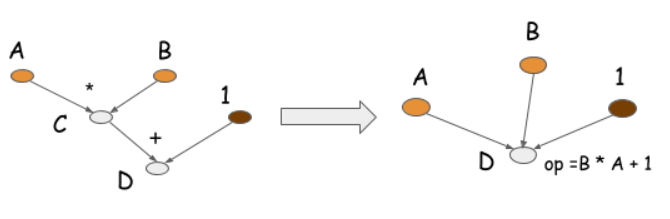# 从一道算法题开始¶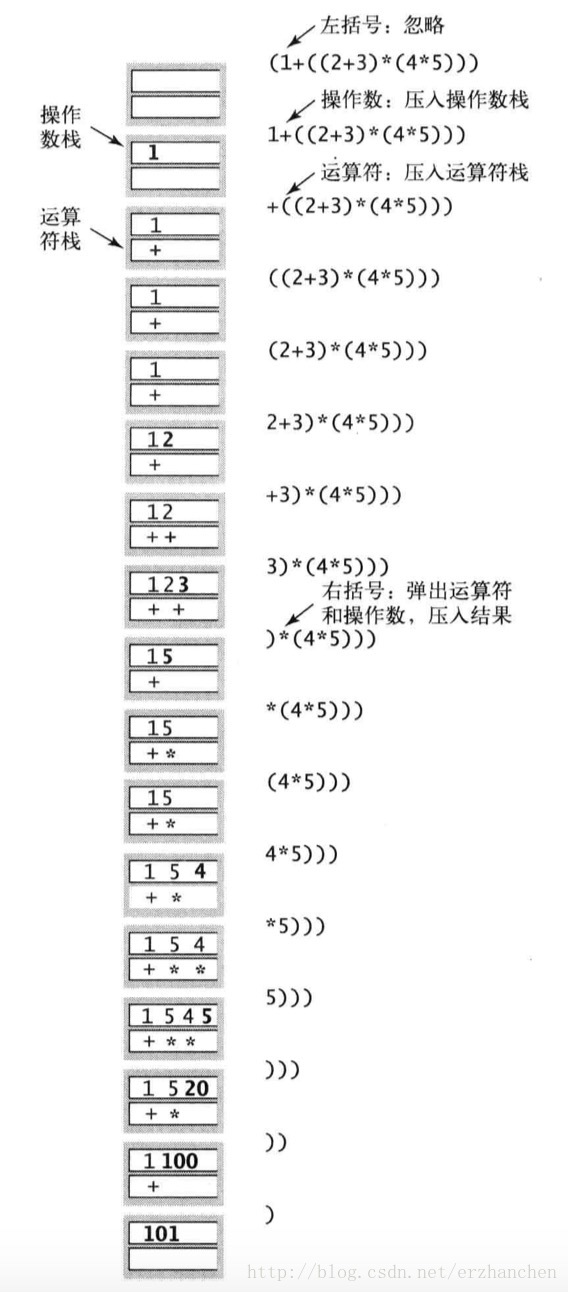# 神经网络里怎么做？¶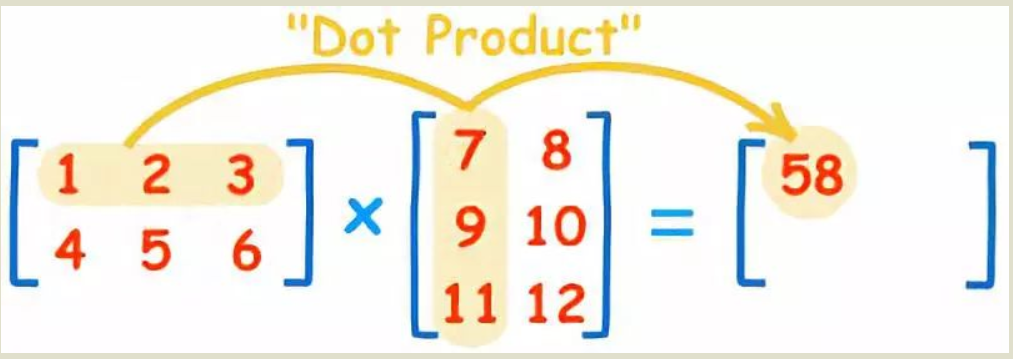Y_1 = W_1*X_1

Y_2 = W_2*Y_1 + B_2 \\ Y_3 = W_3*Y_2 + B_3

Y_3 = W_3*Y_2 \\ Y_3 对W_3求偏导，那就是Y_2， 以此类推

# 计算图¶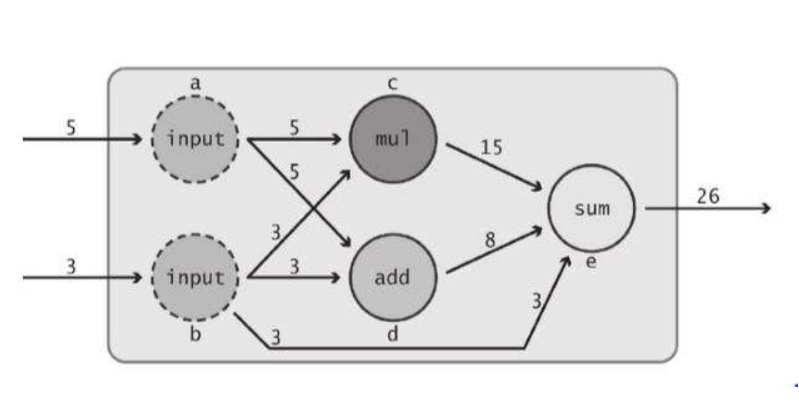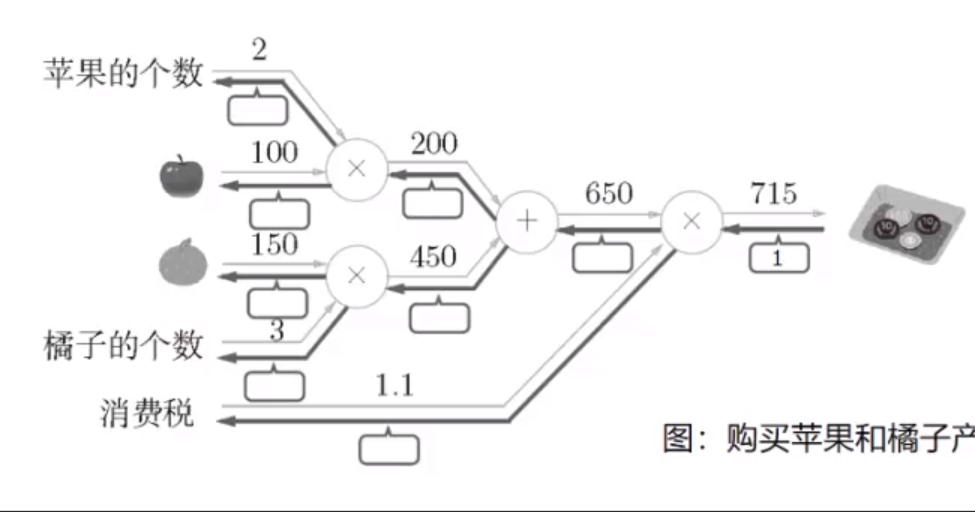650 *1.1 = 715

# 常见的反向传播¶

## 卷积层的反向传播¶

import torch
import torch.nn as nn

conv = nn.Conv2d(in_channels=1, out_channels=1, kernel_size=3, padding=0, bias=False, stride=1)
inputv = torch.range(1, 16).view(1, 1, 4, 4)

print(inputv)
out = conv(inputv)
print(out)
out = out.mean()
out.backward()


tensor([[[[ 3.5000,  4.5000,  5.5000],
[ 7.5000,  8.5000,  9.5000],
[11.5000, 12.5000, 13.5000]]]])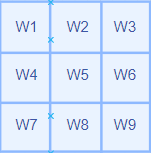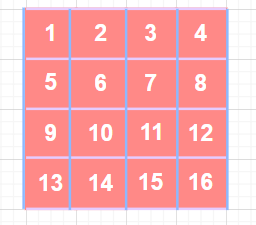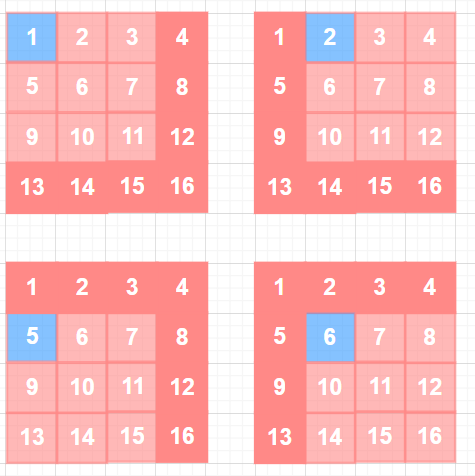1/4 *(1 + 2 + 5 + 6) = 3.5

### 卷积层求导的延申¶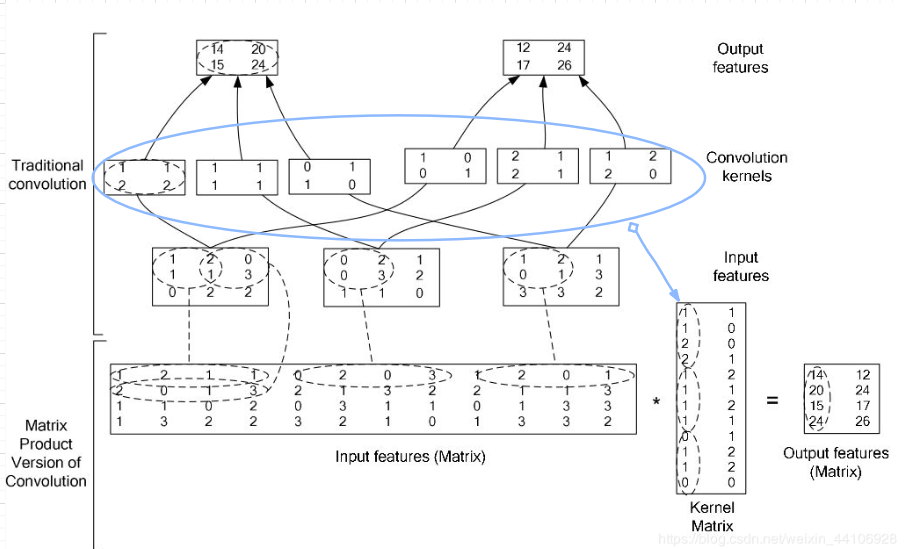## 池化层的反向传播¶

import torch
import numpy as np

inputv = np.array(
[
[1, 2, 3, 4],
[5, 6, 7, 8],
[9, 10, 11, 12],
[13, 14, 15, 16],
]
)
inputv = inputv.astype(np.float)
inputv = inputv.unsqueeze(0)

print(inputv)
pool = torch.nn.functional.max_pool2d(inputv, kernel_size=(3, 3), stride=1)
print(pool)
pool = torch.mean(pool)
print(pool)
pool.backward()


tensor([[[0.0000, 0.0000, 0.0000, 0.0000],
[0.0000, 0.0000, 0.0000, 0.0000],
[0.0000, 0.0000, 0.2500, 0.2500],
[0.0000, 0.0000, 0.2500, 0.2500]]])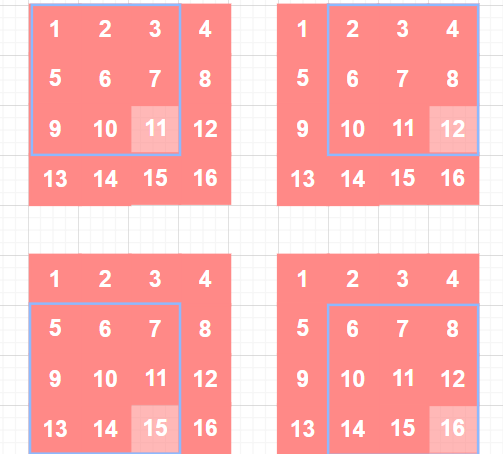1 ➗ 4 = 0.25

# 静态图与动态图的区别¶

## 静态图¶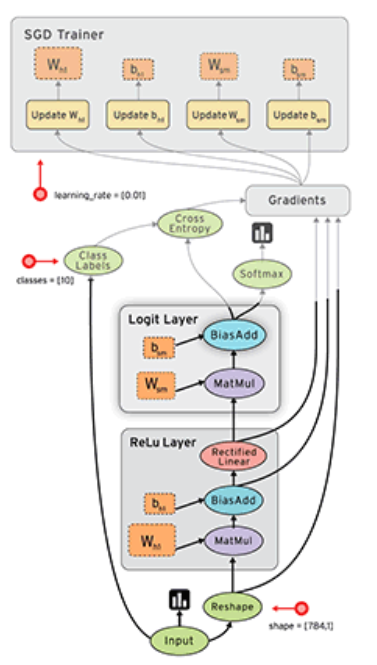tensorflow提出了一个概念，叫PlaceHolder，即数据占位符。PlaceHolder只是有shape，dtype等基础信息，没有实际的数据。在网络定义好后，需要对其进行编译。于是网络就根据每一步骤的placeholder信息进行编译构图，构图过程中检查是否有维度不匹配等错误。待构图好后，再喂入数据给流图。 静态图只构图一次，运行效率也会相对较高点。当然现在的各大框架也在努力优化动态图，缩小两者之间效率差距。

## 两者区别¶

1. 静态图只构图一次
2. 动态图每次运行都重新构图
3. 静态图能在编译中做更好的优化，但动态图的优化也在不断提升中